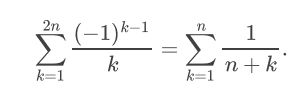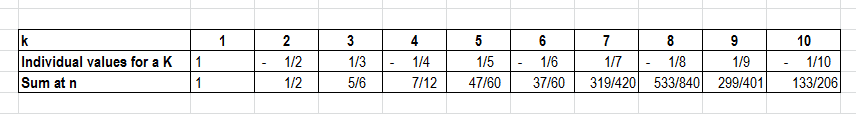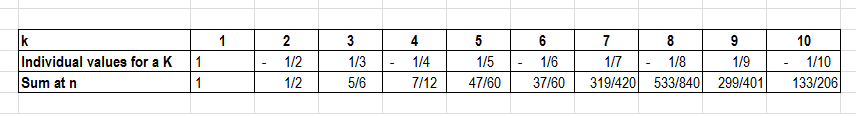# How to solve summation equations with different indices

musicgold
Homework Statement:
I need to prove the equation below. What scares me is the fact that the LHS and RHS have different summation indices.
Relevant Equations:
See belowTo analyze the LHS of this equation, I used (k-1) , k and (K+1) to get

## \frac {(-1)^{k-1} } { (k-1)} \ . \frac {(-1)^k} { (k)} \ . \frac {(-1)^{k+1} } { (k+1)} \ ##

Nothing cancels out in these terms and the sign of each term is the opposite of the previous term.

I calculated the LHS for a k = 1, 2, 3, 4, 5, 6 . The sum is = 1 -1/2 + 1/3 -1/4 + 1/5 -1/6. It looks like an oscillating sum that is going towards 2/3.

How can I proceed from here to get to the RHS?

Thanks

Homework Helper
Hi,

the fact that the LHS and RHS have different summation indices.
they don't: on both sides ##k## is the summation index.

To analyze the LHS of this equation, I used (k-1) , k and (K+1) to get

## \frac {(-1)^{k-1} } { (k-1)} \ . \frac {(-1)^k} { (k)} \ . \frac {(-1)^{k+1} } { (k+1)} \ ##

Nothing cancels out in these terms
I don't see what you are doing here ...

I calculated the LHS for a k = 1, 2, 3, 4, 5, 6 . The sum is = 1 -1/2 + 1/3 -1/4 + 1/5 -1/6
So here you wrote out (but did not add up) the LHS for ##n=3##

Now do the same for the RHS -- I get 37/60

Finally, recognize the pattern and see that an increase in ##n## increases both sides by the same amount.

musicgold
...

Now do the same for the RHS -- I get 37/60

Finally, recognize the pattern and see that an increase in ##n## increases both sides by the same amount.

I am trying to get to the RHS part of the original equation. I don't know how to change the summation index. I think it involves removing half the terms.

Homework Helper
There is no need to change anything. ##n## is the 'independent variable': for each value of ##n##, het LHS gives a value for the sum, and so does the RHS.

So: for ##n=3## you have an LHS value, now fill in ##n=3## on the RHS and evaluate the sum

Homework Helper
I am trying to get to the RHS part of the original equation. I don't know how to change the summation index. I think it involves removing half the terms.

Precisely. It's not that there are "different summation indices". It's that there are twice as many terms on the left as on the right.

There is one term on the right corresponding to every two terms on the left.

Does that give you a hint what you might do?

I don't know what you mean by using k-1, k and k + 1. I'd suggest instead starting with n = 1. That gives you two terms on the left, one on the right. Write out all the terms explicitly. See if you can make that work.

Then try n = 2. That gives you four terms on the left but you already know the sum of the first two. Write out all the terms again, without summation notation.

•Delta2
musicgold
There is no need to change anything. ##n## is the 'independent variable': for each value of ##n##, het LHS gives a value for the sum, and so does the RHS.

So: for ##n=3## you have an LHS value, now fill in ##n=3## on the RHS and evaluate the sum
I think I did not explain my question enough. Let's assume we don't know the RHS part and we are trying to simplify the LHS.

Here is what I am getting when I calculate values for different k and n values. How can I move ahead from here?I don't know what you mean by using k-1, k and k + 1. I'd suggest instead starting with n = 1. That gives you two terms on the left, one on the right. Write out all the terms explicitly. See if you can make that work.

I am using k-1, k, and k+1 in the LHS part to see if there is a pattern.

•Delta2
Staff Emeritus
Homework Helper
Gold Member
I think I did not explain my question enough. Let's assume we don't know the RHS part and we are trying to simplify the LHS.

Here is what I am getting when I calculate values for different k and n values. How can I move ahead from here?I am using k-1, k, and k+1 in the LHS part to see if there is a pattern.
Combine pairs of terms.

The terms for k being odd are: ##\dfrac 1 {1}, \dfrac 1 {3}, \dfrac 1 {5}, \dfrac 1 {7}, \dots , \dfrac 1 {2n-1}## . There are n of these. You can write a general term for these as ##\dfrac 1 {2j-1}## , where j takes on values from 1 to n. Right?

The terms for k being even are: ##-\dfrac 1 {2}, -\dfrac 1 {4}, -\dfrac 1 {6}, -\dfrac 1 {8}, \dots , - \dfrac 1 {2n}## . There are also n of these. Can you you come up with an expression for a general term for these using an index (Why not also use j ?) which takes on values from 1 to n ?

You should be able to include the two expressions in a single summation, then use algebra to combine the two into a single expression.

By the way: The this post only addresses the LHS .

Last edited:
•Delta2
Homework Helper
I worked with n=5 and n=6. I manually obtained the terms and summed to show the expression true for n=5. For n=6 , we will add (1/11 - 1/12) = 1/132 for the expression on the left. For the expression on the right, we will add two terms (1/11 + 1/12) but will loose one - ie 1/(5+1). Then 1/11+1/12-1/6=1/132 also.

You could do something similar with n=m and n=m+1 and hence prove by induction.

•SammyS
Staff Emeritus
Homework Helper
Gold Member
Here is what I am getting when I calculate values for different k and n values. How can I move ahead from here?To make discussion less clumsy, let's define ##L_k ## to be ##\dfrac{(-1)^{(k-1)}}{k} ## .

There is a fundamental problem with your table. You don't sum ##L_k ## from k = 1 to k = n. You sum it from k=1 to k = 2n. The only meaningful entries in the bottom row are for even values of k.

For n = 2, the sum is 7/12.

For n = 3, the sum is 37/60.

Notice that the difference between these is 1/30.

Homework Helper
I am using k-1, k, and k+1 in the LHS part to see if there is a pattern
You don't want that. For a given value of ##n## LHS sums up to something, so does RHS. ##k## has nothing to do with it.

If you now increase ##n## by 1 to ##n+1## , LHS(n+1) = LHS(n) + ##{1\over 2n+1} - {1\over 2n+2}##

and unfortunately RHS(n+1) ##\ne## RHS(n) + ##{1\over (n+1) + (n+1)} ## but, as @neilparker62 indicated
RHS(n+1) = RHS(n) + ##{1\over 2n+1 } + {1\over 2n+2 } - {1\over n+1 }##

If we want the increments to be the same, we must show that $${1\over 2n+1} - {1\over 2n+2} = {1\over 2n+1 } + {1\over 2n+2 } - {1\over n+1 }$$which is not so hard ... 😉

•Delta2